## Payment Processing...# Reasoning Ability

Here we are providing new series of Reasoning Questions for upcoming exams, so the aspirants can practice it on a daily basis.

Study the following information carefully and answer the questions given below.

Nine boxes R, S, T, U, V, W, X, Y and Z are kept one above another in a stack, but not necessarily in the same order. Consecutive alphabetically named boxes are not kept adjacent to each other.

Box S and box V are kept adjacent to each other. Only one box is kept between box S and box U. Box U is kept four boxes above box R. Only two boxes are kept between box R and box Y. As many boxes kept between X and V is same as between Y and X which is kept immediately above box T. Box Z is kept two boxes above box W.

1) Which of the following box is kept immediately below box R?

A.The box which is kept immediately above box U

B.The box which is kept two boxes below box T

C.The box which is kept three boxes above box X

D.The box which is kept four boxes below box W

E.None of these

2) Four of the following five are alike in a certain way as per the given arrangement and hence form a group, find the one that doesn’t belong to that group.

A.Z-S

B.W-R

C.T-V

D.U-V

E.R-Y

3) If all the boxes are arranged according to reverse alphabetic order from the top to bottom, then which of the following boxes remain unchanged in their position?

A.Z

B.W

C.V

D.Both (a) and (c)

E.Both (a) and (b)

4) How many boxes are kept between box S and the one which is kept immediately below box X?

A.Three

B.Five

C.Four

D.One

E.None of these

5) What is the position of box U with respect to the box W?

A.Three boxes below

B.Immediately below

C.Two boxes above

D.Immediately above

E.None of these

Explanation :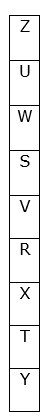We have,

• Box S and box V are kept adjacent to each other.

From the above condition, there are two possibilities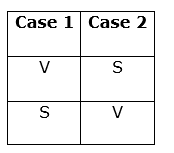Again we have,

• Only one box is kept between box S and box U.
• Box U is kept four boxes above box R.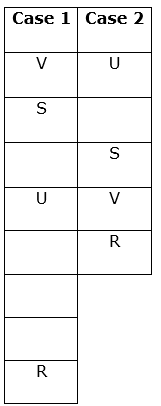Again we have,

• Only two boxes are kept between box R and box Y.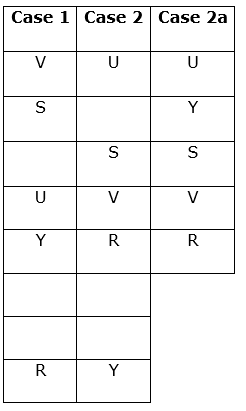Again we have,

• As many boxes are kept between X and V as between Y and X which is kept immediately above box T.

So Case 1 and Case 2a get eliminated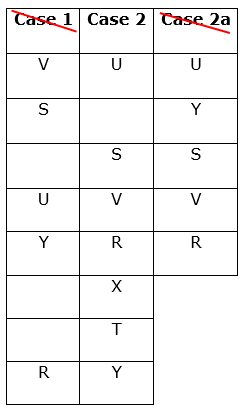Again we have,

• Box Z is kept two boxes above box W.

Hence Case2 shows the final arrangement